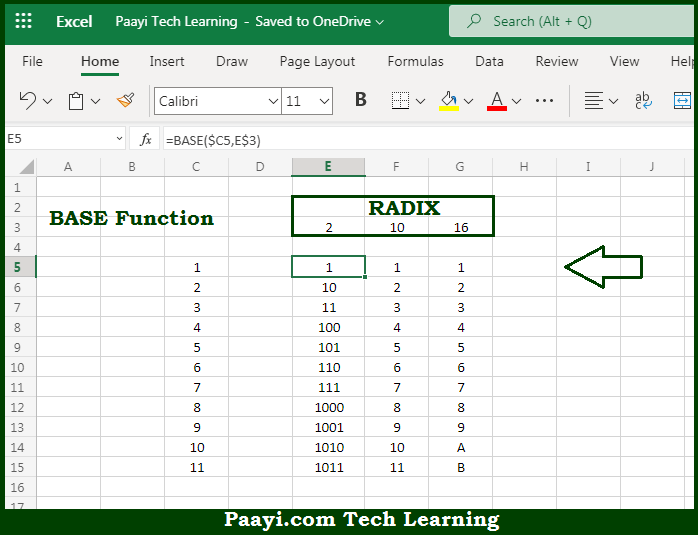# Learn How to Use Microsoft Excel BASE Function

Written by | 0 Comments | 522 Views

In this article, you will learn how to use the Microsoft Excel BASE function and its prime function in Microsoft Excel. You will also get to know the Microsoft Excel BASE function return value and syntax with the help of some examples.

Microsoft Excel BASE Function

The main function of the Microsoft Excel BASE function is to convert numbers to another base. So, with the help of the BASE function, you can able to convert the number to the text representation of the given radix (base). So, with the help of the Microsoft Excel BASE function, you can easily convert the number to another base.

Return Value of BASE Function

The return value will be the text representation of another number.

Syntax of BASE Function

Where the arguments:

• number: This is the number you want to convert into another representation.
• radix: This is the base of the number being converted to.
• min-length: It guarantees the minimum length of the string returned by padding the string with zeros (optional).

How to Use Microsoft Excel BASE Function?Microsoft Excel BASE function is used to convert numbers to another base. So, with the help of the BASE function, you can able to convert the number to the text representation of the given radix (base). The radix value specifies the base representation of the returned text. So, with the help of the Microsoft Excel BASE function, you can easily convert the number to another base.